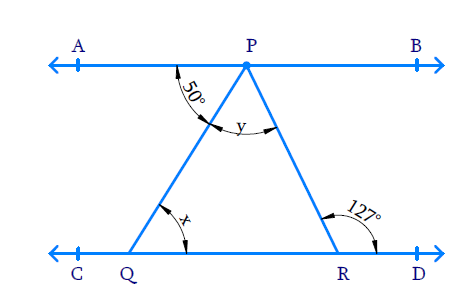# Ex.6.2 Q5 Lines and Angles Solution - NCERT Maths Class 9

Go back to  'Ex.6.2'

## Question

In the given figure, if $$AB \| CD,\;\; \angle APQ = 50 ^ { \circ }$$ and $$\angle PRD = 127 ^ { \circ }$$, find $$x$$ and $$y$$.Video Solution
Lines And Angles
Ex 6.2 | Question 5

## Text Solution

What is known?

$$AB \| CD,\;\; \angle APQ = 50 ^ { \circ }$$ and $$\angle PRD = 127 ^ { \circ }$$

What is unknown?

$$x =?$$ and $$y = ?$$

Reasoning:

• When a ray intersects a line, sum of adjacent angles formed is $$180^ {\circ}$$.
• When two parallel lines are cut by a transversal, alternate interior angles formed are equal.

Steps:

$$AB$$ and $$CD$$ are parallel lines cut by transversal $$PQ$$ hence the alternate interior angles formed are equal.

$$\angle APQ = \angle PQR$$ and hence $$x = 50^ {\circ}$$.

Similarly, $$AB$$ and $$CD$$ are parallel lines cut by transversal $$PR$$ hence the alternate angles formed are equal.

\begin{align} \angle APR+ \angle PRD &= 127 ^ { 0 } \\ \angle APQ + \angle QPR &= \angle PRD \\&= 127 ^ { \circ } \\ 50 ^ { \circ } + y &= 127 ^ { 0 } \\ y &= (127 ^ { 0 } - 50 ^ { \circ }) \\ y &= 77 ^ { \circ } \end{align}

Learn from the best math teachers and top your exams

• Live one on one classroom and doubt clearing
• Practice worksheets in and after class for conceptual clarity
• Personalized curriculum to keep up with school